Next: Dynamical (Chiral) Symmetry Breaking Up: QUANTUM FIELDS OF NUCLEAR Previous: Quantum Chromodynamics (QCD)

Chiral Symmetry and the Isospin

We now proceed with the program outlined at the end of the previous section, namely, knowing from experiment that mesons exist we begin by introducing the relevant degrees of freedom. We also know that meson is a complicated solution of the QCD quark and gluon fields that involve a real quark-antiquark pair. However, without ever being able to find this solution, let us try to identify basic features of the meson that result from the underlying QCD structure.

Let us concentrate on a small piece of the QCD Lagrangian density (21), i.e., on the up and down quark components of the middle term, i.e.,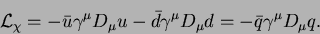(25)

The gluon fields and the color SU(3) matrices are not essential now, so we have hidden all that in the SU(3) covariant derivative: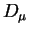=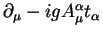. On the other hand, we have explicitly indicated the up and down quark fields,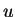and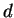, and moreover, we have combined both fields into the quark iso-spinor,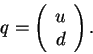(26)

To be specific,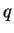contains 24 components, i.e., two quarks, each in three colors, and each built as a four-component Dirac spinor. However, the Dirac and color structure is again not essential, so in the present section we may think aboutas two-component spinor. For a moment we have also disregarded the quark mass terms - we reinsert them slightly below.

What is essential now are the symmetry properties of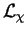. This piece of the Lagrangian density looks like a scalar in the two-component field, i.e., it is manifestly invariant with respect to unitary mixing of up and down quarks. We formalize this observation by introducing the isospin Pauli matrices,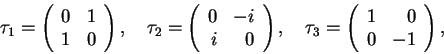(27)

and we introduce the unitary mixing of up and down quarks in the language of rotations in the abstract isospin space. And yes, this is exactly the same iso-space that we know very well from nuclear structure physics, where the upper and lower components are the neutron and proton. We come back to that later.

What is slightly less obvious, but in fact trivial to anybody acquainted with the relativistic Lorentz group, is the fact thatis also invariant with respect to multiplying the quark fields by the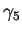Dirac matrix shown in Eq. (18). This property results immediately from the commutation properties of the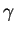matrices (remember that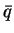=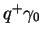). So in fact, we have altogether six symmetry generators of, namely,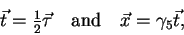(28)

where the arrows denote vectors in the iso-space.

It is now easy to identify the symmetry group of. We introduce the left-handed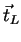and right-handed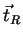generators,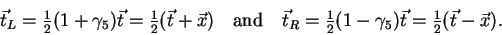(29)

Since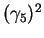=1, they fulfill the following commutation relations: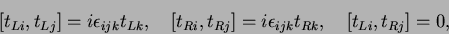(30)

i.e.,generates the SU(2) group,generates another SU(2) group, and since they commute with one another, the complete symmetry group is SU(2)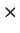SU(2). We call this group chiral.

This result is quite embarrassing, because it is in a flagrant disagreement with experiment. On the one hand, we know very well that particles appear in iso-multiplets. For example, there are two nucleons, a neutron and a proton, that can be considered as upper and lower components of an iso-spinor, and there are three pions,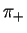,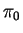, and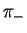, that can be grouped into an iso-vector. So there is no doubt that there is an isospin SU(2) symmetry in Nature, but, on the other hand, what about the second SU(2) group? In the Lorentz group, theDirac matrix changes the parity of the field, so ifwas really a symmetry then particles should appear in pairs of species having opposite parities. This is not so in our world. Nucleons have positive intrinsic parity, and their negative-parity brothers or sisters are nowhere to be seen. Parity of pions is negative, and again, the positive-parity mirror particles do not exist any near the same mass.

So the Nature tells us that the SU(2)SU(2) symmetry of the QCD Lagrangian must be dynamically broken. It means that the Lagrangian has this symmetry, while the physical solutions do not. We already learned that these physical solutions are very complicated, and we are unable to find them and check what are their symmetries. But we do not really need that - experiment tells us that chiral symmetry must be broken, and hence, we can built theories that incorporate this feature on a higher level of description.

Before we construct a model in which the dynamical symmetry breaking mechanism is explicitly built in (and before we show explicitly what such a symmetry breaking really is), let us first reinsert the quark-mass terms into the discussed piece of the Lagrangian: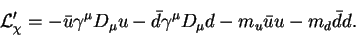(31)

By a simple calculation we can now easily show that neither of the two mass terms, nor any linear combination thereof, are invariant with respect to the chiral group SU(2)SU(2). For certain, had the quark masses been equal, the two combined mass terms would have constituted an isoscalar (an invariant with respect to the isospin group), but even then they would not be chiral scalars (invariants with respect to the chiral group). So the non-zero quark masses break the chiral symmetry. What are the values of these masses has to be taken from the experiment, and indeed, neither the up and down quark masses are zero, nor they are equal to one another, see Fig. 1. The chiral symmetry is therefore broken in two ways: (i) explicitly, by the presence of a symmetry breaking term in the Lagrangian, and (ii) dynamically, as discussed above. Without going into details, we just mention that the non-zero mass of the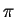mesons results from the non-zero quark masses, see Ref. Wei99, chap. 19.3. For more quark flavors taken into account, the dimensionality of the chiral group increases, i.e., when three quarks,, and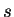are considered the chiral group is SU(3)SU(3).

That is about this far that we can move forward by using the QCD quark Lagrangian. We have identified basic symmetry properties of the QCD solutions, and now we have to go to the next level of description, namely, consider composite objects built of quarks. This way of proceeding is called the effective field theory (EFT). We do not build fields of composite objects from the lower-level fields. Instead, we consider the composite objects to be elementary, and we guess their properties from symmetry considerations of the lower-level fields; otherwise, it would have been too difficult a task. Before we arrive at sufficiently high energies, or small distances, at which the internal structure of composite objects becomes apparent, we can safely live without knowing exactly how the composite objects are constructed.Next: Dynamical (Chiral) Symmetry Breaking Up: QUANTUM FIELDS OF NUCLEAR Previous: Quantum Chromodynamics (QCD)
Jacek Dobaczewski 2003-01-27